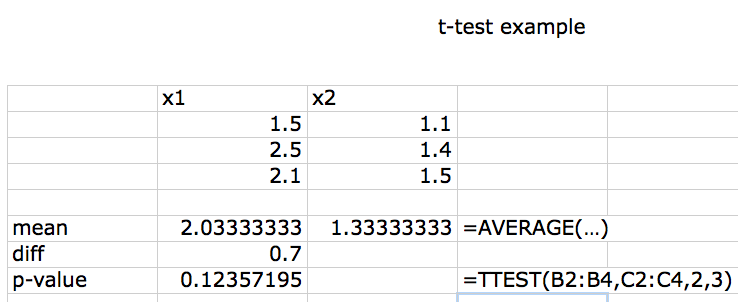10 November 2015

## outline

• experimental design
• data exploration
• statistical tests & assumptions
• analysis platforms

source code## the most important thing

• Design your experiment well and execute it well:
• Don't: you're doomed - statistics can't save you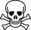• "To consult the statistician after an experiment is finished is often merely to ask him to conduct a post mortem examination. He can perhaps say what the experiment died of." (Fisher)
• "The combination of some data and an aching desire for an answer does not ensure that a reasonable answer can be extracted from a given body of data." (Tukey)
• randomization, replication, control

## randomization

• random assignment to treatments
• poorer alternative: haphazard assignment
• stratification
(i.e., randomize within groups)
• related: experimental blinding

## replication

• how big does your experiment need to be?
• power: probability of detecting an effect of a particular size,
if one exists
• more generally: how much information? what kinds of mistakes? (Gelman & Carlin, 2014)
• underpowered studies
• failure is likely
• cheating is likely
• significance filter $$\to$$ biased estimates
• overpowered studies waste time, lives, \$
• pseudoreplication (Davies & Gray, 2015; Hurlbert, 1984) confounding sampling units with treatment units

## power analysis

• need to guess effect size and variability
• biological common sense
• previous studies
• OK to simplify design (e.g. ANOVA $$\to$$ $$t$$-test)
• methods
apropos("^power")  ## base-R functions
library("sos"); findFn("{power analysis}")

## control

• maximize desired variation
• e.g. large doses (tradeoff with biological realism)
• minimize undesired variation
• within-subjects designs
• e.g. paired, randomized-block, crossover
• minimize environmental variation
• e.g. environmental chambers;
clonal or inbred lines
• isolate desired effects: positive/negative controls
(vehicle-only, cage treatments, etc.)
• control for variation statistically (e.g. ANCOVA)

## descriptive statistics

• check that values are reasonable
• categorical
• tabulation, cross-tabulation
• univariate
• mean, standard deviation
• median, minimum, maximum
• bivariate
• correlations

## graphics

• univariate
• histogram
• grouped
• box-and-whisker (violin)
• bivariate
• scatterplots
• multivariate
• scatterplot matrices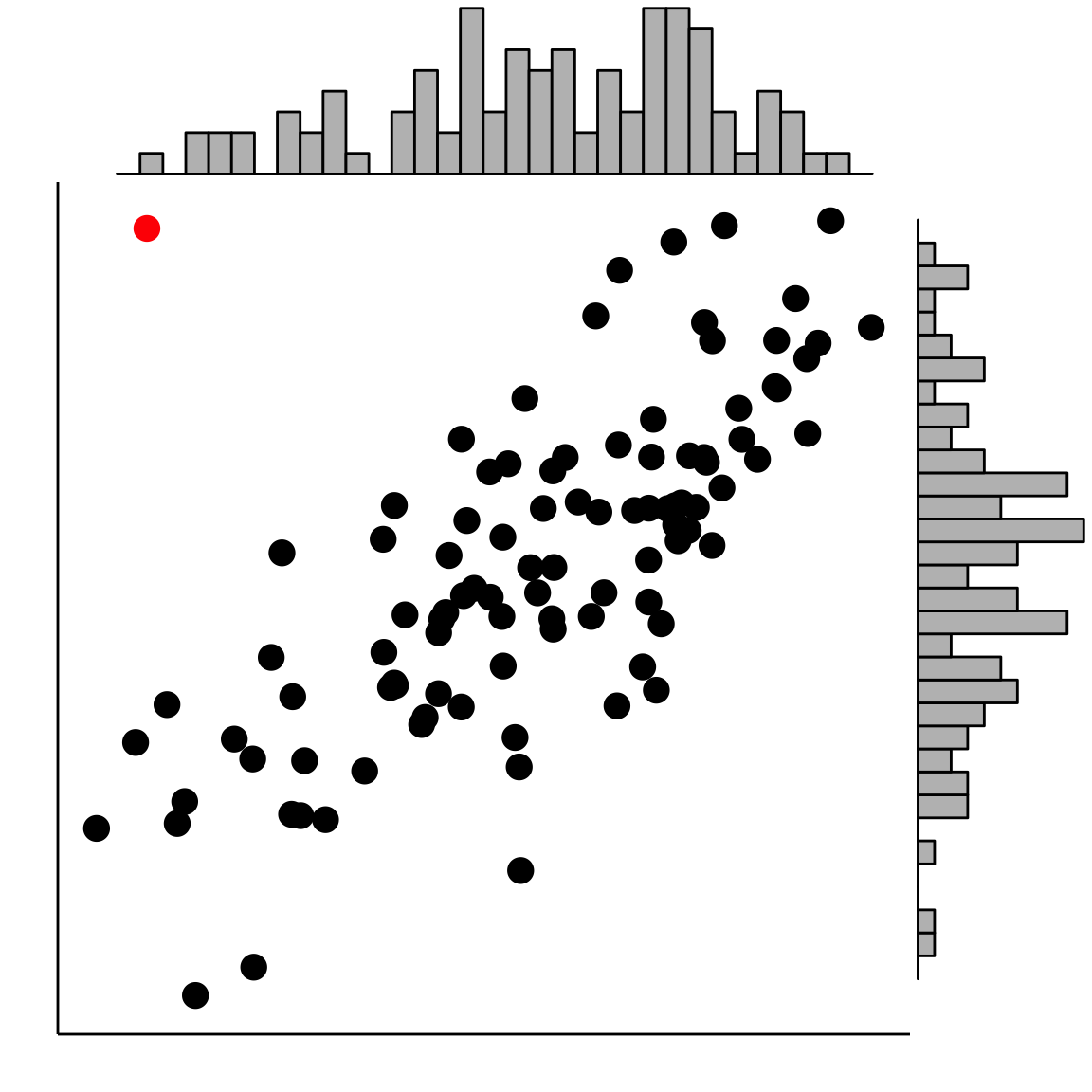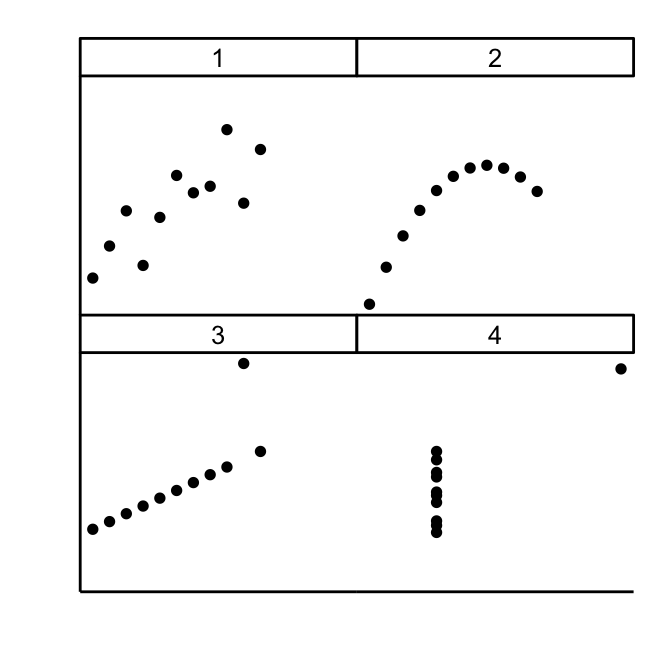## assumptions

• independence (hard to test!)
• homogeneity of variance (vs. heteroscedasticity)
• linearity
• Normality (least important)
• outliers; skew; "fat tails" (Student, 1927)
• distributional assumptions apply to the conditional distribution of the response variable## diagnostics

• hypothesis tests are not generally appropriate:
• graphical diagnostics
• residuals plots (linearity, heteroscedasticity)
• influence plots (outliers)
• Q-Q plots (Normality)
• Box-Cox plots (transformations)

## diagnostics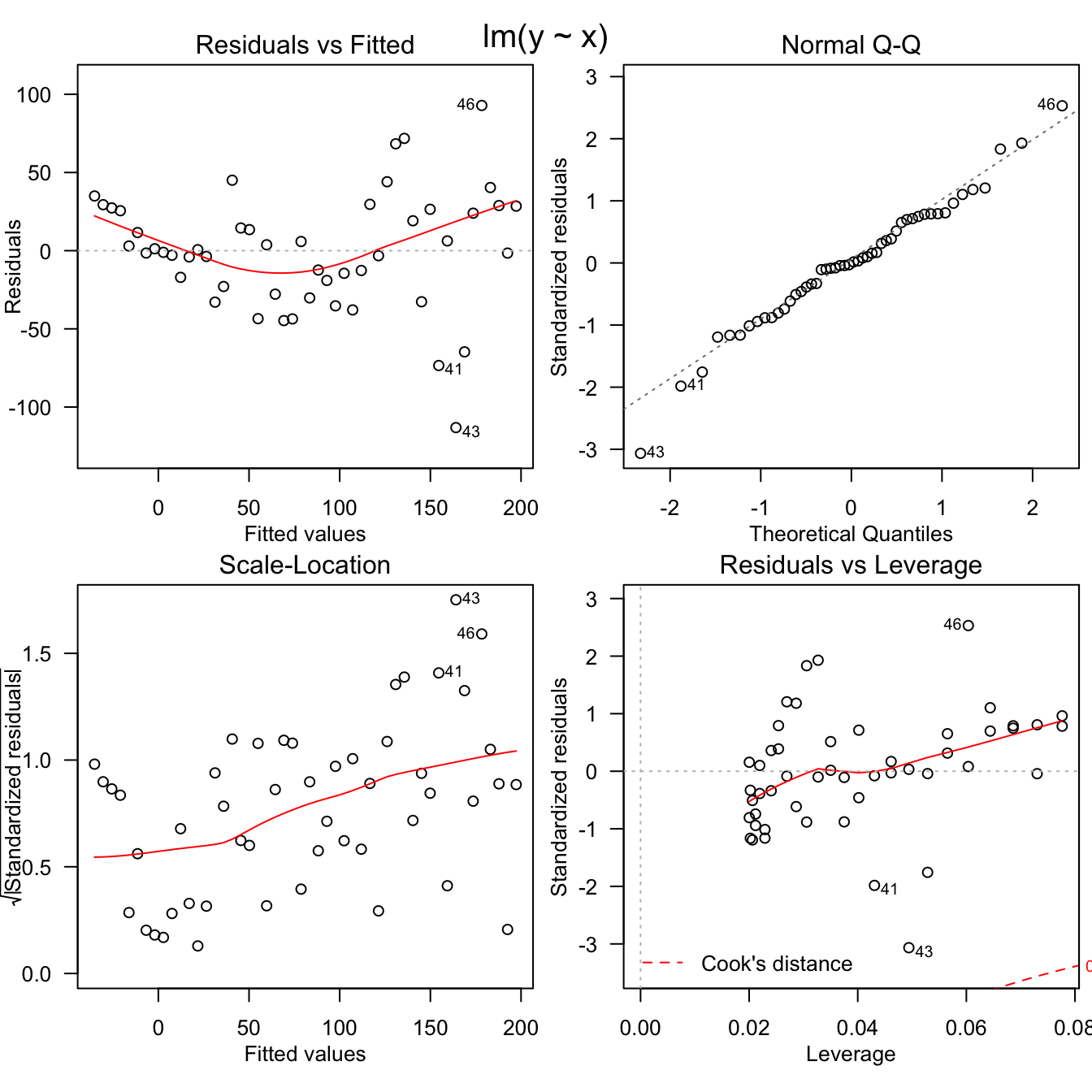## (the data set)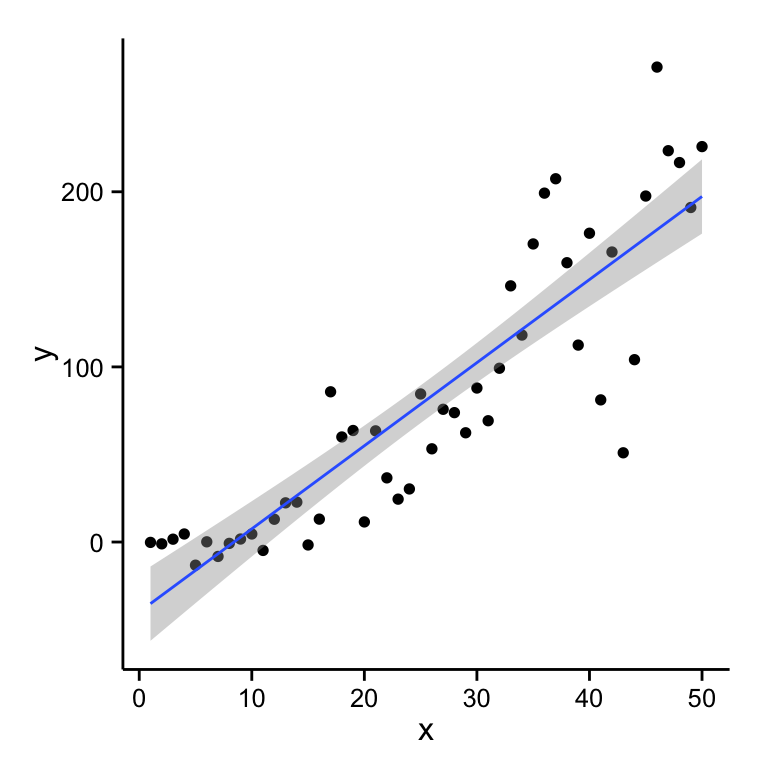## dealing with violations

• drop outliers (report both analyses)
• transform (e.g. log transform: Box-Cox analysis)
• non-parametric (rank-based) tests
(e.g. Mann-Whitney-Wilcoxon, Kruskal-Wallis)
• relax assumptions/do fancier stats, e.g.
• logistic regression (0/1 outcomes)

## what should you use?

• try to connect scientific & statistical questions
• data type
• see decision tree or table
• if your question doesn't fit in this tree,
think about how much you like statistics …
• nonparametric stats
• slight loss of power
• stronger assumptions than you think
• $$p$$-values only - no effect size

## interpretation

• focus on effect sizes/CIs
• eschew vacuous hypothesis statements
• don't accept the null hypothesis
• "the difference between significant and non-significant is not significant" (Gelman & Stern, 2006)
• avoid snooping/$$p$$-hacking (Simmons et al., 2011):
preregister your analyses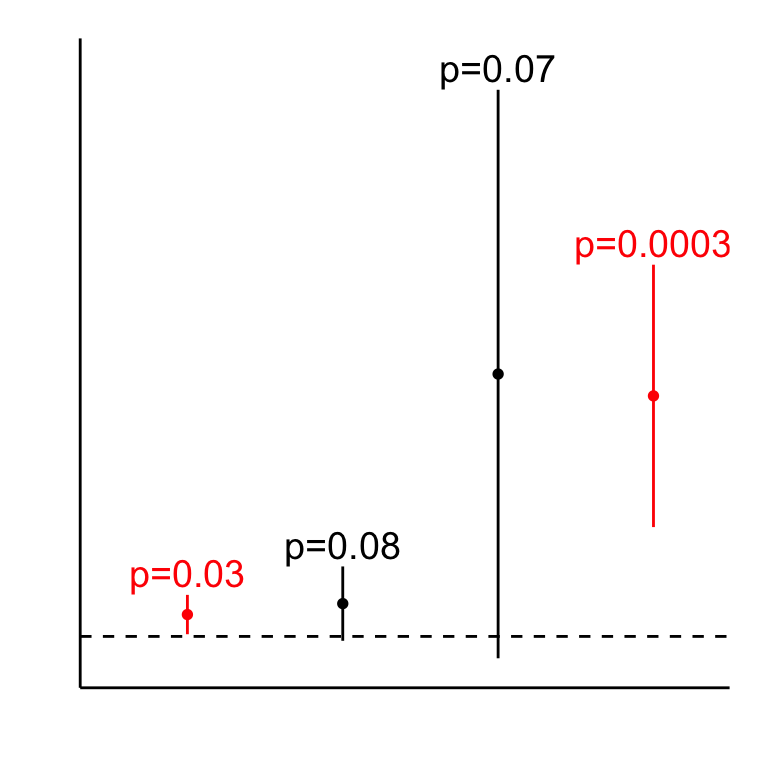## Criteria

• simple/weak vs. complex/powerful
• GUI vs command-line
• default: use what your lab uses

## Excel

• ubiquitous
• open alternatives (Open Office)
• data in plain sight
• good enough for simple stuff
• occasional traps (McCullough & Heiser, 2008)
• archive your data as CSV, not XLSX

## stats packages

• SPSS, JMP, SAS, …
• more reliable than Excel
• more powerful than Excel
• point & click (mostly)

## R

• powerful; free & open
• reproducible: script-based
• hardest to learn
• R Commander if you need a GUI
• great for data manipulation, graphics
(once you learn how)

Using this analogy programs like SPSS are busses, easy to use for the standard things, but very frustrating if you want to do something that is not already preprogrammed.

R is a 4-wheel drive SUV (though environmentally friendly) with a bike on the back, a kayak on top, good walking and running shoes in the passenger seat, and mountain climbing and spelunking gear in the back. R can take you anywhere you want to go if you take time to learn how to use the equipment, but that is going to take longer than learning where the bus stops are in SPSS.

Greg Snow, R-help (May 2006)

## t-test (R)

x1 = c(1.5,2.5,2.1)
x2 = c(1.1,1.4,1.5)
t.test(x1,x2)
##
##  Welch Two Sample t-test
##
## data:  x1 and x2
## t = 2.226, df = 2.6648, p-value = 0.1236
## alternative hypothesis: true difference in means is not equal to 0
## 95 percent confidence interval:
##  -0.3759079  1.7759079
## sample estimates:
## mean of x mean of y
##  2.033333  1.333333

## t-test (Excel)